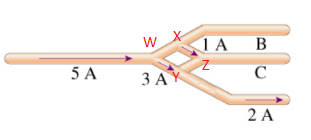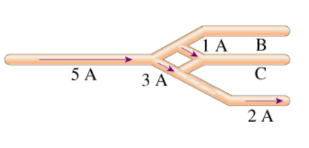# Problem: The currents through several segments of a wire object are shown in (Figure 1).Part A. What is the magnitude of the current Ic in segment C?Part B. What is the direction of the current Ic in segment C?

###### FREE Expert Solution(1)

Applying Kirchoff's junction rule at junction Y, we have:

3 A = 2 A + iYZ

iYZ = 3 A - 2 A = 1 A

99% (141 ratings)###### Problem Details

The currents through several segments of a wire object are shown in (Figure 1).Part A. What is the magnitude of the current Ic in segment C?

Part B. What is the direction of the current Ic in segment C?